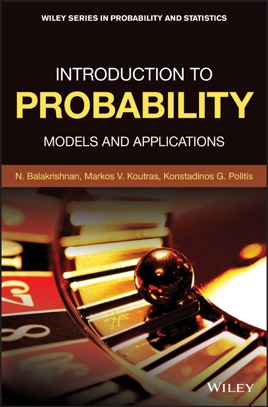• \$134.99

## Publisher Description

An essential guide to the concepts of probability theory that puts the focus on models and applications

Introduction to Probability offers an authoritative text that presents the main ideas and concepts, as well as the theoretical background, models, and applications of probability. The authors—noted experts in the   field—include a review of problems where probabilistic models naturally arise, and discuss the methodology to tackle these problems.

A wide-range of topics are covered that include the concepts of probability and conditional probability, univariate discrete distributions, univariate continuous distributions, along with a detailed presentation of the most important probability distributions used in practice, with their main properties and applications.

Designed as a useful guide, the text contains theory of probability, de finitions, charts, examples with solutions, illustrations, self-assessment exercises, computational exercises, problems and a glossary. This important text:

• Includes classroom-tested problems and solutions to probability exercises
• Highlights real-world exercises designed to make clear the concepts presented
• Uses Mathematica software to illustrate the text’s computer exercises
• Features applications representing worldwide situations and processes
• Offers two types of self-assessment exercises at the end of each chapter, so that students may review the material in that chapter and monitor their progress.

Written for students majoring in statistics, engineering, operations research, computer science, physics, and mathematics, Introduction to Probability: Models and Applications is an accessible text that explores the basic concepts of probability and includes detailed information on models and applications.